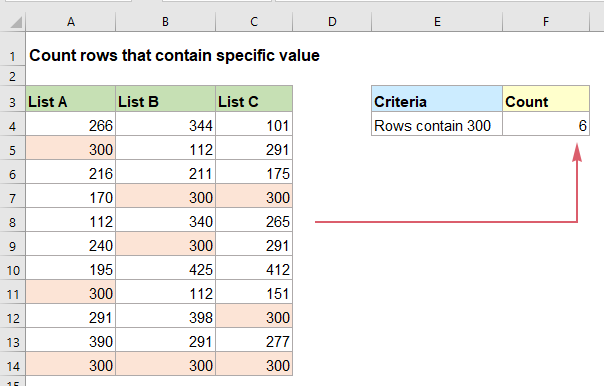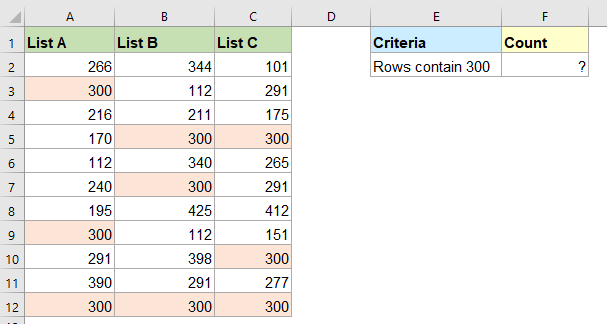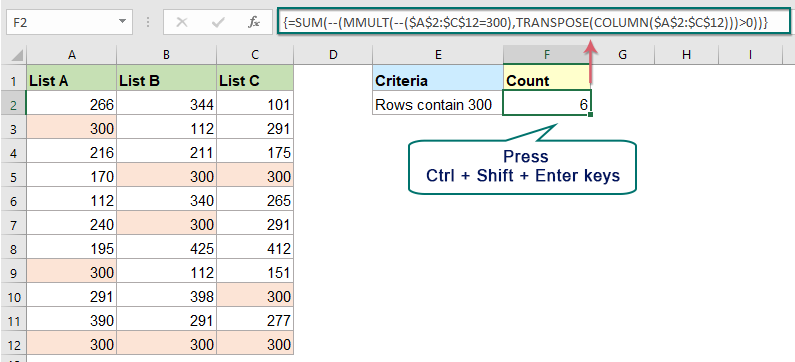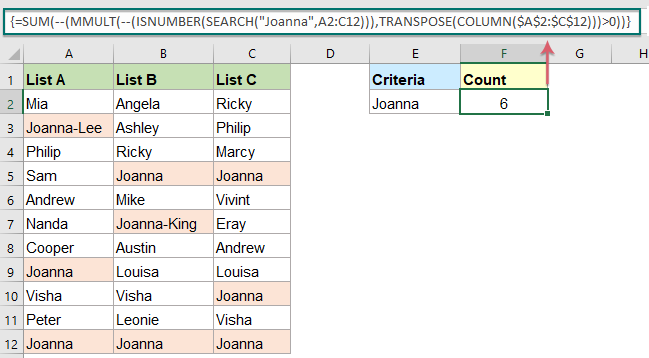## Count number of rows that contain specific values in Excel

It may be easy for us to count the number of cells with specific value in an Excel worksheet. However, to get the number of rows that contain specific values could be rather complicated. In this case, a more complex formula based on the SUM, MMULT, TRANSPOSE and COLUMN functions may do you a favor. This tutorial will talk about how to create this formula to deal with this job in excel.#### Count number of rows that contain specific values

For instance, you have a range of values in a worksheet, and now, you need to count the number of rows with a given value “300” as below screenshot shown:To get the number of rows that contain specific values, the generic syntax is:

{=SUM(–(MMULT(–(data=X),TRANSPOSE(COLUMN(data)))>0))}
Array formula, should press Ctrl + Shift + Enter keys together.
• data: The range of cells to check if contain the specific value;
• X: The specific value you use to count the rows.

1. Please enter or copy the below formula into a blank cell where you want to put the result:

=SUM(--(MMULT(--(\$A\$2:\$C\$12=300),TRANSPOSE(COLUMN(\$A\$2:\$C\$12)))>0))

2. Then press Ctrl + Shift + Enter keys together to get the correct result, see screenshot:##### Explanation of the formula:

=SUM(--(MMULT(--(\$A\$2:\$C\$12=300),TRANSPOSE(COLUMN(\$A\$2:\$C\$12)))>0))

• --\$A\$2:\$C\$12=300: This expression checks if the value “300” exists in the range A2:C12, and will generate a TRUE and False array result. The double negative sign is used to convert the TRUEs to 1s, and FALSEs to 0s. So, you will get the result as this: {0,0,0;1,0,0;0,0,0;0,1,1;0,0,0;0,1,0;0,0,0;1,0,0;0,0,1;0,0,0;1,1,1}. The array consisting of 11 rows and 3 columns will work as the array1 in the MMULT function.
• TRANSPOSE(COLUMN(\$A\$2:\$C\$12)): The COLUMN function here is used to get the column number of the range A2:C12, it returns a 3-column array like this: {1,2,3}. And then the TRANSPOSE function swaps this array to a 3-row array {1;2;3}, functioning as the array2 within the MMULT function.
• MMULT(--(\$A\$2:\$C\$12="Joanna"),TRANSPOSE(COLUMN(\$A\$2:\$C\$12))): This MMULT function returns the matrix product of the above two arrays, you will get the result as this: {0;1;0;5;0;2;0;1;3;0;6}.
• SUM(--(MMULT(--(\$A\$2:\$C\$12="Joanna"),TRANSPOSE(COLUMN(\$A\$2:\$C\$12)))>0))= SUM(--{0;1;0;5;0;2;0;1;3;0;6}>0): First, check for the values in the array greater than 0: If a value is greater than 0, a TRUE is displayed; if less than 0, a FALSE is displayed. And then the double negative sign forces the TRUEs and FALSEs to be 1s and 0s, so you will get this: SUM({0;1;0;1;0;1;0;1;1;0;1}). Finally, the SUM function sums the values in the array to return the result: 6.

Tips:

If you need to count the number of rows that contain a specific text in a worksheet, please apply the below formula, and remember to press the Ctrl + Shift + Enter keys together to get the total count:

=SUM(--(MMULT(--(ISNUMBER(SEARCH("Joanna",A2:C12))),TRANSPOSE(COLUMN(\$A\$2:\$C\$12)))>0))#### Relative function used:

• SUM:
• The Excel SUM function returns the sum of values supplied.
• MMULT:
• The Excel MMULT function returns the matrix product of two arrays.
• TRANSPOSE:
• The TRANSPOSE function will return an array in a new orientation based on a certain range of cells.
• COLUMN:
• The COLUMN function returns the number of column which formula appears or returns the column number of given reference.

#### More articles:

• Count Rows If Meet Internal Criteria
• Supposing, you have a report of product sales of this year and last year, and now, you may need to count products where the sales in this year are greater than last year, or the sales in this year are less than last year as below screenshot shown. Normally, you can add a helper column for calculating the sale difference between the two years, and then use COUNTIF to get result. But, this article, I will introduce the SUMPRODUCT function to get the result directly without any helper column.
• Count Rows If Meet Multiple Criteria
• Count the number of rows in a range based on multiple criteria, some of which depends on the logical tests that work at the row-level, the SUMPRODUCT function in Excel may do you a favor.
• Count Number Of Cells Equals To One Of Many Values
• Supposing, I have a list of products in column A, now, I want to get the total number of specific products Apple, Grape and Lemon which listed in range C4:C6 from column A as below screenshot shown. Normally, in Excel, the simple COUNTIF and COUNTIFS functions will not work in this scenario. This article, I will talk about how to solve this job quickly and easily with the combination of SUMPRODUCT and COUNTIF functions.

### The Best Office Productivity Tools

#### Kutools for Excel - Helps You To Stand Out From Crowd

 Popular Features: Find, Highlight or Identify Duplicates  |  Delete Blank Rows  |  Combine Columns or Cells without Losing Data  |  Round without Formula ... Super VLookup: Multiple Criteria  |  Multiple Value  |  Across Multi-Sheets  |  Fuzzy Lookup... Adv. Drop-down List: Easy Drop Down List  |  Dependent Drop Down List  |  Multi-select Drop Down List... Column Manager: Add a Specific Number of Columns  |  Move Columns  |  Toggle Visibility Status of Hidden Columns  |  Compare Columns to Select Same & Different Cells ... Featured Features: Grid Focus  |  Design View  |  Big Formula Bar  |  Workbook & Sheet Manager | Resource Library (Auto Text)  |  Date Picker  |  Combine Worksheets  |  Encrypt/Decrypt Cells  |  Send Emails by List  |  Super Filter  |  Special Filter (filter bold/italic/strikethrough...) ... Top 15 Toolsets:  12 Text Tools (Add Text, Remove Characters ...)  |  50+ Chart Types (Gantt Chart ...)  |  40+ Practical Formulas (Calculate age based on birthday ...)  |  19 Insertion Tools (Insert QR Code, Insert Picture from Path ...)  |  12 Conversion Tools (Numbers to Words, Currency Conversion ...)  |  7 Merge & Split Tools (Advanced Combine Rows, Split Excel Cells ...)  |  ... and more

Kutools for Excel Boasts Over 300 Features, Ensuring That What You Need is Just A Click Away...#### Office Tab - Enable Tabbed Reading and Editing in Microsoft Office (include Excel)

• One second to switch between dozens of open documents!
• Reduce hundreds of mouse clicks for you every day, say goodbye to mouse hand.
• Increases your productivity by 50% when viewing and editing multiple documents.
• Brings Efficient Tabs to Office (include Excel), Just Like Chrome, Edge and Firefox.